## SINE: 一种基于扩散模型的单图像编辑解决方案

Title: <SINE: SINgle Image Editing with Text-to-Image Diffusion Models>

Paper: https://arxiv.org/pdf/2212.04489.pdf

Github: https://github.com/zhang-zx/SINE.git

## 导读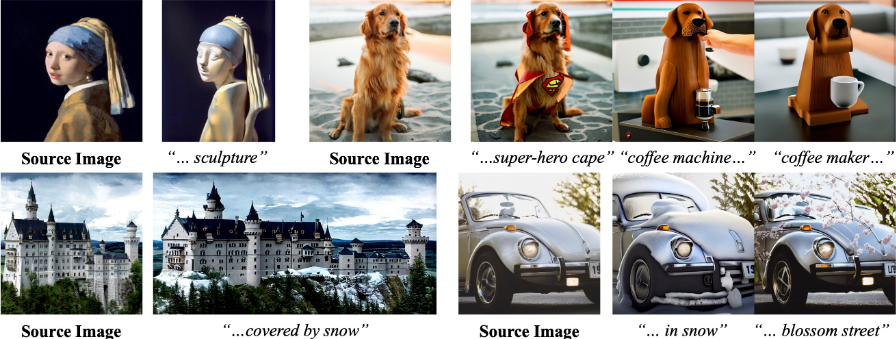## 总览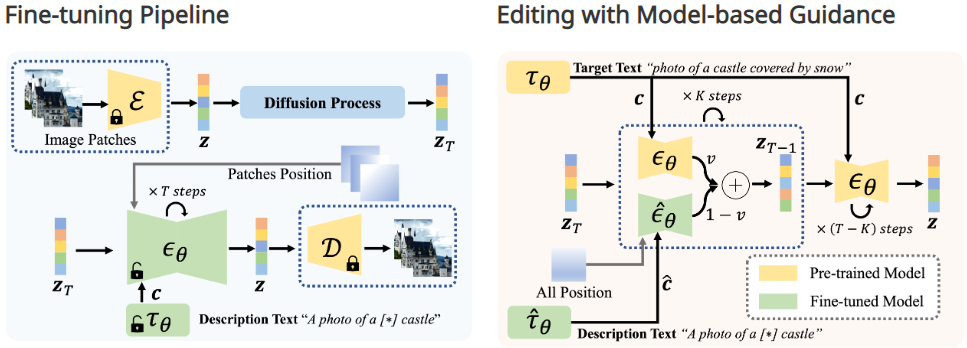## 实验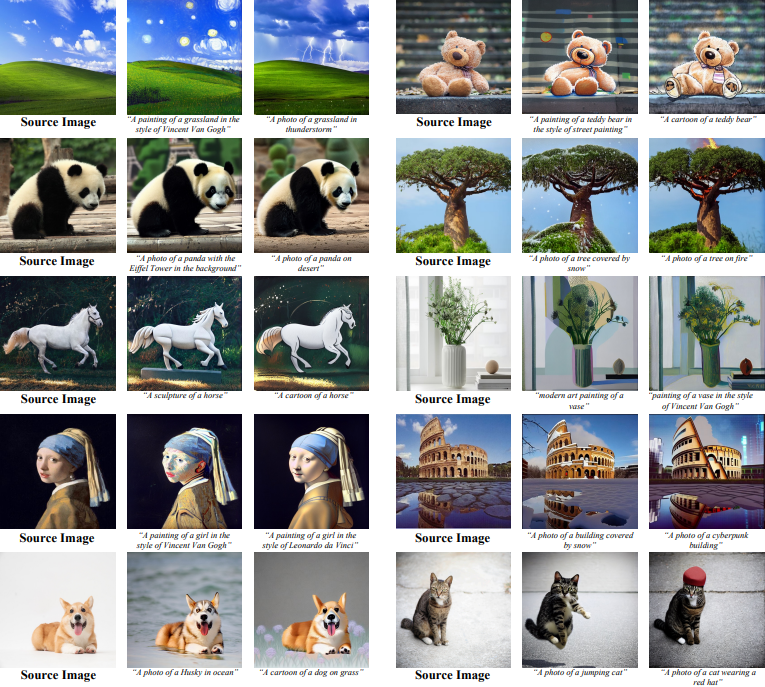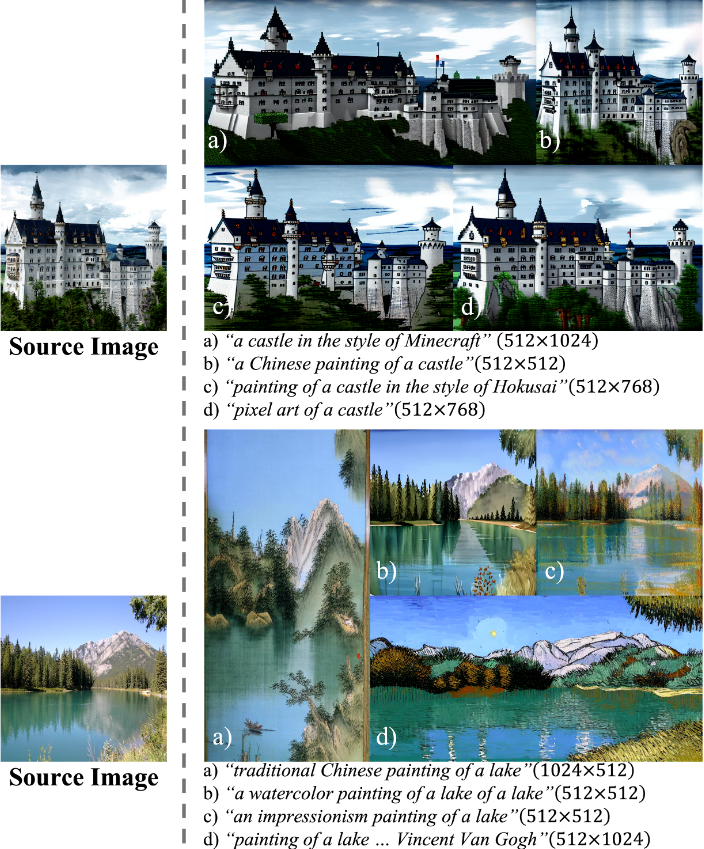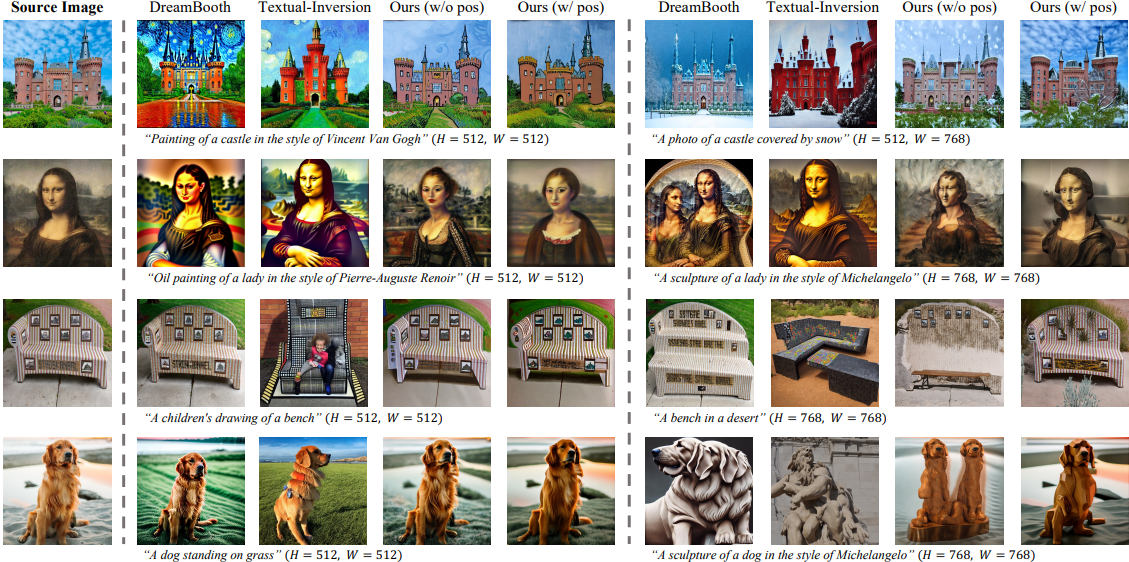## 应用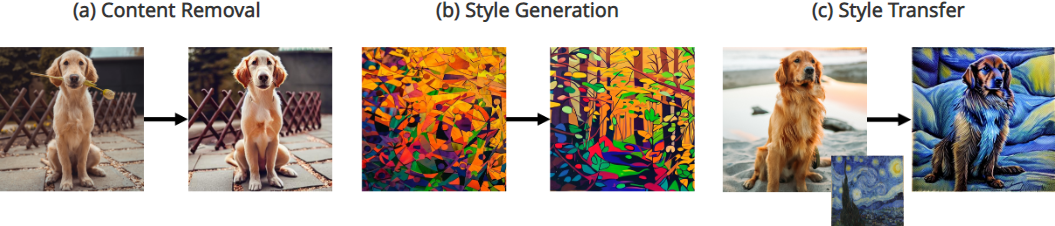The generation resolution is set to H = 768 and W = 1024. We use K = 400 and v = 0.7 in this sample.The output resolution is set to H = 768 and W = 1024. We use K = 400 and v = 0.65 in this example.The output resolution is set to H = 768 and W = 1024. We use K = 400 and v = 0.7 in this example.The output resolution is set to H = 768 and W = 1024. We use K = 500 and v = 0.8 in this example.The output resolution is set to H = 768 and W = 1024. We use K = 500 and v = 0.8 in this example.The output resolution is set to H = 1024 and W = 768. We use K = 400 and v = 0.6 in this example.## 总结

• 使用基于`补丁`微调方法对预先训练的模型进行微调，直到它过拟合单个图像。
• 在采样时间，使用过拟合的模型来指导预先训练的`扩散模型`进行图像合成，这既保证了结果的保真度，又利用了预先训练模型的泛化能力